Showing first {{hits.length}} results of {{hits_total}} for {{searchQueryText}}{{hits.length}} results for {{searchQueryText}}

No Search Results

Feynman diagrams provide a very compact and intuitive way of representing interactions between particles. These diagrams can be included into LaTeX documents thanks to a few packages. One of the older packages is feynmf which uses MetaPost in order to generate the diagrams. More recently, a new package called TikZ-Feynman has been published which uses TikZ in order to generate Feynman diagrams. Contents 1 TikZ-Feynman 1.1 Loading the Package 1.2 A First Diagram 1.3 Adding Styles 1.4 When the Algorithm Isn't Enough 1.4.1 Invisible Edges 1.4.2 Alternative Algorithms 1.4.3 Manual Placement 2 FeynMF 2.1 Introduction 2.2 Vertices 2.3 Labels 2.4 Line styles 3 Further Reading TikZ-Feynman   TikZ-Feynman Template in Overleaf TikZ-Feynman is a LaTeX package allowing Feynman diagrams to be easily generated within LaTeX with minimal user instructions and without the need of external programs. It builds upon the TikZ package and its graph drawing algorithms in order to automate the placement of many vertices. TikZ-Feynman still allows fine-tuned placement of vertices so that even complex diagrams can be generated with ease. The most up-to-date information for TikZ-Feynman will always be on the project page and in the package documentation on CTAN. Loading the Package After installing the package, the TikZ-Feynman package can be loaded with \usepackage{tikz-feynman} in the preamble. It is recommend that you also specify the version of TikZ-Feynman to use with the compat package option: \usepackage[compat=1.0.0]{tikz-feynman}. This ensures that any new versions of TikZ-Feynman do not produce any undesirable changes without warning. A First Diagram Feynman diagrams can be declared with the \feynmandiagram command. It is analogous to the \tikz command from TikZ and requires a final semi-colon (;) to finish the environment. For example, a simple s-channel diagram is: \feynmandiagram [horizontal=a to b] { i1 -- [fermion] a -- [fermion] i2, a -- [photon] b, f1 -- [fermion] b -- [fermion] f2, };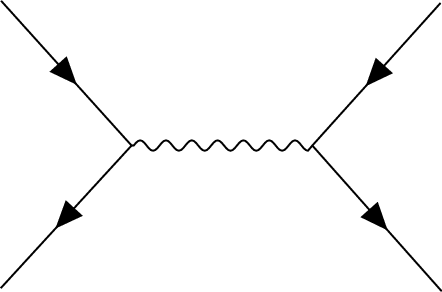Let's go through this example line by line: Line 1 \feynmandiagram introduces the Feynman diagram and allows for optional arguments to be given in the brackets []. In this instance, horizontal=a to b orients the algorithm outputs such that the line through vertices a and b is horizontal. Line 2 The left fermion line is drawn by declaring three vertices (i1, a and i2) and connecting them with edges --. Just like the \feynmandiagram command above, each edge also take optional arguments specified in brackets []. In this instance, we want these edges to have arrows to indicate that they are fermion lines, so we add the fermion style to them. As you will see later on, optional arguments can also be given to the vertices in exactly the same way. Line 3 This edge connects vertices a and b with an edge styled as a photon. Since there is already a vertex labelled a, the algorithm will connect it to a new vertex labeled b. Line 4 This line is analogous to line 2 and introduces two new vertices, f1 and f2. It re-uses the previously labelled b vertex. Line 5 Finish the declaration of the Feynman diagram. The final semi-colon (;) is important. The name given to each vertex in the graph does not matter. So in this example, i1, i2 denote the initial particles; f1, f2 denotes the final particles; and a, b are the end points of the propagator. The only important aspect is that what we called a in line 2 is also a in line 3 so that the underlying algorithm treats them as the same vertex. The order in which vertices are declared does not matter as the default algorithm re-arranges everything. For example, one might prefer to draw the fermion lines all at once, as with the following example (note also that the way we named vertices is completely different): \feynmandiagram [horizontal=f2 to f3] { f1 -- [fermion] f2 -- [fermion] f3 -- [fermion] f4, f2 -- [photon] p1, f3 -- [photon] p2, };As a final remark, the calculation of where vertices should be placed is usually done through an algorithm written in Lua. As a result, LuaTeX is required in order to make use of these algorithms. If LuaTeX is not used, TikZ-Feynman will default to a more rudimentary algorithm and will warn the user instead. Adding Styles So far, the examples have only used the photon and fermion styles. The TikZ-Feynman package comes with quite a few extra styles for edges and vertices which are all documented over in the package documentation. For example, it is possible to add momentum arrows with momentum=, and in the case of end vertices, the particle can be labelled with particle=. To demonstrate how they are used, we take the generic s-channel diagram from earlier and make it a electron-positron pairs annihilating into muons: \feynmandiagram [horizontal=a to b] { i1 [particle=$$e^{-}$$] -- [fermion] a -- [fermion] i2 [particle=$$e^{+}$$], a -- [photon, edge label=$$\gamma$$, momentum'=$$k$$] b, f1 [particle=$$\mu^{+}$$] -- [fermion] b -- [fermion] f2 [particle=$$\mu^{-}$$], };In addition to the style keys documented below, style keys from TikZ can be used as well: \feynmandiagram [horizontal=a to b] { i1 [particle=$$e^{-}$$] -- [fermion, very thick] a -- [fermion, opacity=0.2] i2 [particle=$$e^{+}$$], a -- [red, photon, edge label=$$\gamma$$, momentum'={[arrow style=red]$$k$$}] b, f1 [particle=$$\mu^{+}$$] -- [fermion, opacity=0.2] b -- [fermion, very thick] f2 [particle=$$\mu^{-}$$], };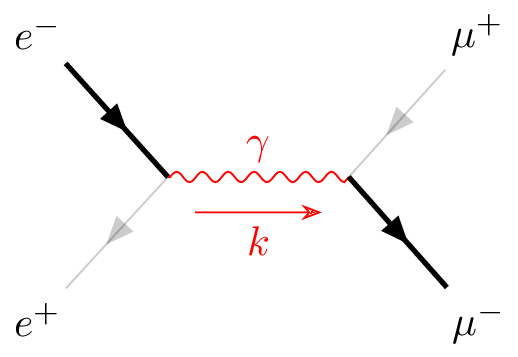For a list of all the various styles that TikZ provides, have a look at the TikZ manual; it is extremely thorough and provides many usage examples. When the Algorithm Isn't Enough By default, the \feynmandiagram and \diagram commands use the spring layout algorithm to place all the edges. The spring layout algorithm attempts to `spread out' the diagram as much as possible which—for most simpler diagrams—gives a satisfactory result; however in some cases, this does not produce the best diagram and this section will look at alternatives. There are three main alternatives: Add invisible edges While still using the default algorithm, it is possible to force certain vertices to be closer together by adding extra edges and making them invisible through draw=none. The algorithm will treat these extra edges in the same way, but they are simply not drawn at the end; Use a different algorithm In some circumstances, other algorithms may be better suited. Some of the other graph layout algorithms are listed in the package documentation, and an exhaustive list of all algorithms and their parameters is given in the TikZ manual; Manual placement As a last resort, very complicated or unusual diagrams will require each vertex to be manually placed. Invisible Edges The underlying algorithm treats all edges in exactly the same way when calculating where to place all the vertices, and the actual drawing of the diagram (after the placements have been calculated) is done separately. Consequently, it is possible to add edges to the algorithm, but prevent them from being drawn by adding draw=none to the edge style. This is particularly useful if you want to ensure that the initial or final states remain closer together than they would have otherwise as illustrated in the following example (note that opacity=0.2 is used instead of draw=none to illustrate where exactly the edge is located). % No invisible to keep the two photons together \feynmandiagram [small, horizontal=a to t1] { a [particle=$$\pi^{0}$$] -- [scalar] t1 -- t2 -- t3 -- t1, t2 -- [photon] p1 [particle=$$\gamma$$], t3 -- [photon] p2 [particle=$$\gamma$$], };% Invisible edge ensures photons are parallel \feynmandiagram [small, horizontal=a to t1] { a [particle=$$\pi^{0}$$] -- [scalar] t1 -- t2 -- t3 -- t1, t2 -- [photon] p1 [particle=$$\gamma$$], t3 -- [photon] p2 [particle=$$\gamma$$], p1 -- [opacity=0.2] p2, };Alternative Algorithms The graph drawing library from TikZ has several different algorithms to position the vertices. By default, \diagram and \feynmandiagram use the spring layout algorithm to place the vertices. The spring layout attempts to spread everything out as much as possible which, in most cases, gives a nice diagram; however, there are certain cases where this does not work. A good example where the spring layout doesn't work are decays where we have the decaying particle on the left and all the daughter particles on the right. % Using the default spring layout \feynmandiagram [horizontal=a to b] { a [particle=$$\mu^{-}$$] -- [fermion] b -- [fermion] f1 [particle=$$\nu_{\mu}$$], b -- [boson, edge label=$$W^{-}$$] c, f2 [particle=$$\overline \nu_{e}$$] -- [fermion] c -- [fermion] f3 [particle=$$e^{-}$$], };% Using the layered layout \feynmandiagram [layered layout, horizontal=a to b] { a [particle=$$\mu^{-}$$] -- [fermion] b -- [fermion] f1 [particle=$$\nu_{\mu}$$], b -- [boson, edge label'=$$W^{-}$$] c, c -- [anti fermion] f2 [particle=$$\overline \nu_{e}$$], c -- [fermion] f3 [particle=$$e^{-}$$], };You may notice that in addition to adding the layered layout style to \feynmandiagram, we also changed the order in which we specify the vertices. This is because the layered layout algorithm does pay attention to the order in which vertices are declared (unlike the default spring layout); as a result, c--f2, c--f3 has a different meaning to f2--c--f3. In the former case, f2 and f3 are both on the layer below c as desired; whilst the latter case places f2 on the layer above c (that, the same layer as where the W-boson originates). Manual Placement In more complicated diagrams, it is quite likely that none of the algorithms work, no matter how many invisible edges are added. In such cases, the vertices have to be placed manually. TikZ-Feynman allows for vertices to be manually placed by using the \vertex command. The \vertex command is available only within the feynman environment (which itself is only available inside a tikzpicture). The feynman environment loads all the relevant styles from TikZ-Feynman and declares additional TikZ-Feynman-specific commands such as \vertex and \diagram. This is inspired from PGFPlots and its use of the axis environment. The \vertex command is very much analogous to the \node command from TikZ, with the notable exception that the vertex contents are optional; that is, you need not have {} at the end. In the case where {} is specified, the vertex automatically is given the particle style, and otherwise it is a usual (zero-sized) vertex. To specify where the vertices go, it is possible to give explicit coordinates though it is probably easiest to use the positioning library from TikZ which allows vertices to be placed relative to existing vertices. By using relative placements, it is possible to easily tweak one part of the graph and everything will adjust accordingly—the alternative being to manually adjust the coordinates of every affected vertex. Finally, once all the vertices have been specified, the \diagram* command is used to specify all the edges. This works in much the same way as \diagram (and also \feynmandiagram), except that it uses an very basic algorithm to place new nodes and allows existing (named) nodes to be included. In order to refer to an existing node, the node must be given in parentheses. This whole process of specifying the nodes and then drawing the edges between them is shown below for the muon decay: \begin{tikzpicture} \begin{feynman} \vertex (a) {$$\mu^{-}$$}; \vertex [right=of a] (b); \vertex [above right=of b] (f1) {$$\nu_{\mu}$$}; \vertex [below right=of b] (c); \vertex [above right=of c] (f2) {$$\overline \nu_{e}$$}; \vertex [below right=of c] (f3) {$$e^{-}$$}; \diagram* { (a) -- [fermion] (b) -- [fermion] (f1), (b) -- [boson, edge label'=$$W^{-}$$] (c), (c) -- [anti fermion] (f2), (c) -- [fermion] (f3), }; \end{feynman} \end{tikzpicture}FeynMF The feynmf package lets you easily draw Feynman diagrams in your LaTeX documents. All you need to do is specify the vertices, the particles and the labels, and it will automatically layout and draw your diagram for you. Introduction Let's start with a quick example: \begin{fmffile*}{diagram} \begin{fmfgraph}(40,25) \fmfleft{i1,i2} \fmfright{o1,o2} \fmf{fermion}{i1,v1,o1} \fmf{fermion}{i2,v2,o2} \fmf{photon}{v1,v2} \end{fmfgraph} \end{fmffile*}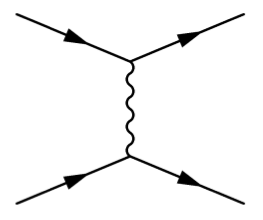Open this example in Overleaf The fmffile* environment must be put around all of your Feynman diagrams. You can use fmffile environment for multiple diagrams, so you can put one around your whole document and forget about it. The second argument to the fmffile environment tells LaTeX where to write the files that it uses to store the diagram. You can name this whatever you want, but you need to run metafont on your diagram between LaTeX runs in order for your diagram to show up (Overleaf does this automatically): pdflatex feynmf.tex mf '\mode:=laserjet; input diagram' pdflatex feynmf.tex The 'fmfgraph' environment starts a Feynman diagram, and the figures in brackets afterwards specify the width and height of the diagram. Vertices The first thing you need to do is specify your external vertices, and where they should be positioned. You can name your vertices anything you like, and say where they should be positioned with the commands \fmfleft, \fmfright, \fmftop, \fmfbottom. For example % Creates two vertices on the left called i1 and i2 \fmfleft{i1,i2} % Creates two vertices on the right called o1 and o2 \fmfright{o1,o2} You can connect up vertices with the \fmf, which will create new vertices if you pass in names that haven't been created yet. For example % Will create a fermion line between i1 and % the newly created v1, and between v1 and o1. \fmf{fermion}{i1,v1,o1} % Will create a photon line between v1 and the newly created v2 \fmf{photon}{v2,v2} Labels A vertex can be labelled using the \fmflabel command, which takes two arguments: the label to apply to the vertex, and the name of the vertex to apply it to. For example, in the above diagram, if we add in the following labels, we get the updated diagram below: \fmflabel{$v_1$}{v1} \fmflabel{$v_2$}{v2}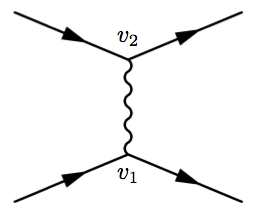Open this example in Overleaf Note that math mode can used inside the vertex labels, as we have done above. Line styles We've seen the 'photon' and 'fermion' line styles above, but the feynmf package support many more. Appearance Name(s)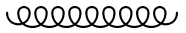gluon, curly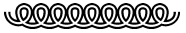dbl_curly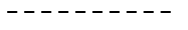dashes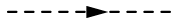scalar, dashes_arrow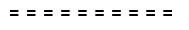dbl_dashes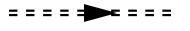dbl_dashes_arrow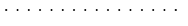dots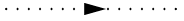ghost, dots_arrow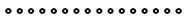dbl_dots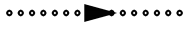dbl_dots_arrow phantom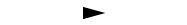phantom_arrow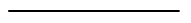vanilla, plain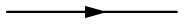fermion, electron, quark, plain_arrow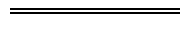double, dbl_plain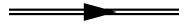double_arrow, heavy, dbl_plain_arrow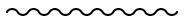boson, photon, wiggly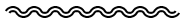dbl_wiggly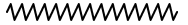zigzag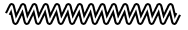dbl_zigzag Further Reading For more information see: Chemistry formulae Molecular orbital diagrams TikZ package Drawing Diagrams Directly in LaTeX Inserting Images List of Greek letters and math symbols The feynmf package documentation.